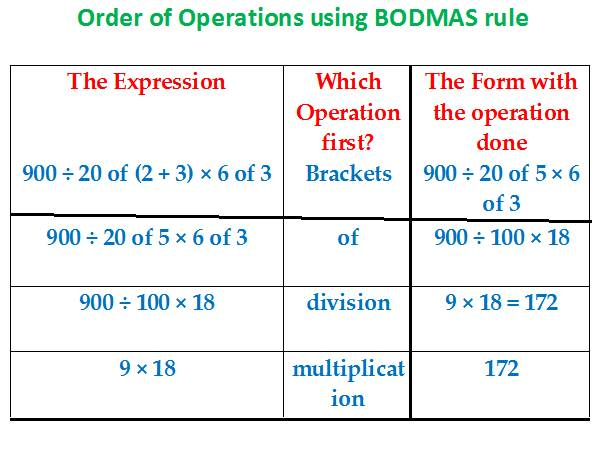## Simplification

1. What does the word “of” signify?

“of” denotes multiplication.

Examples:

• One-fifth of 5 is = (1/5) × 5 = 1,
• 50% of 100 = (50/100) × 100 = ½

2. The Rule on “of”

The operation (multiplication) denoted by “of” is first simplified before division and multiplication.

Example 1:

• 100 ÷ 4 of 5 = 100 ÷ 4 × 5 = 100 ÷ 20 = 5

3. Division is simplified before multiplication

Example 2:

• 100 ÷ 4 of 5 × 2

= 100 ÷ 20 × 2

Now, which should you simplify first in 100 ÷ 20 × 2?

“Division in 100 ÷ 20” Or “Multiplication in 20 × 2”?

Answer: Always Division first, Multiplication Next.

So, 100 ÷ 20 × 2 = 5 × 2 = 10

4. Brackets are simplified first before Division or Multiplication.

Example 4:

• Simplify: 900 ÷ 20 of (2 + 3) × 6 of 39900 ÷ 20 of (2 + 3) × 6 of 3 =

900 ÷ 20 of 5 × 6 of 3 =

900 ÷ 100 × 18 =

9 × 18 = 172

Rules of Operations on Brackets:

• Simplify “—“first before opening brackets.
• 4 + (3 – 2) = 4 + 1 = 5
• When there is a minus sign before brackets, then multiply it to the number obtained after opening up the brackets.
• 5 – (3 – 2) = 5 – 1 = 4
• 5 – (3 – 4) = 5 – (-1) = 5 + 1 = 6
• Simplify “( )” before “{ }”

{- 2 – (- 3)} × 4 =

{- 2 + 3} × 4 =

{1} × 4 =

1× 4 = 4

• Simplify “{ }” before “[ ]”

100 – [90 – {80 – (70 – 60 – 50)}] =

100 – [90 – {80 – (- 40)}] =

100 – [90 – {80 + 40}] =

100 – [90 – 120] =

100 – [- 30] =

100 + 30 = 130

Let us summarize all of the above rules of operations into one Mnemonic as BODMAS for Order of Operation”

BODMAS stands for Bracket Of Division, Multiplication, Addition, Subtraction.

The BODMAS Rule resolves the conflict between two different operations when they come up for simultaneous application.

The rule tells that the operation denoted by its letter coming first in BODMAS will take place before the other operation denoted by its letter coming later on in BODMAS.

In BODMAS, the operation denoted by a letter precedes that denoted by the latter letter.

Let us apply one by one each of the operations separately and combinedly in the last example below

• 4 + 2 – 3 = 6 – 3 = 3

In BODMAS, the letter A comes before S. Therefore, Addition precedes Subtraction.

• 4 + 2 × 3 = 4 + 6 = 10

In BODMAS, M precedes A, so, Multiplication first, Addition next.

• 4 ÷ 2 × 3 = 2 × 3 = 6

In BODMAS, D precedes M, so Division first, Multiplication next.

• 4 of 6 ÷ 2 = 4 × 6 ÷ 2 = 24 ÷ 2 = 12

In BODMAS, “O” stands for “of”, and “ of” denotes multiplication. And, O precedes D, therefore perform the Operation of multiplication denoted by “of” before Division.

• (100 ÷ 5) of 2

In BODMAS, B precedes O.

So, whichever operation B encloses shall precede the operation denoted by O, i.e. multiplication.

In (100 ÷ 5) of 2, the brackets enclose the division operation.

So, division (as enclosed by brackets) is performed before multiplication (as denoted by “of”)

(100 ÷ 5) of 2 = 20 × 2 = 40